Do you like the article?
Share it with others -

Use new possibilities of MetaTrader 5

13 September 2018, 14:02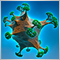0
17 832

### Introduction

In the previous article, I demonstrated how to quickly develop the EA graphical interface (GUI). Here we will connect the already developed GUI with the EA functionality.

### Getting symbol and indicator data

First, we need to arrange getting symbol and indicator data. Let's present forex symbols as a table relying on the filter values in the Symbols filter input field. This is done by the CProgram::GetSymbols() method.

At the beginning of the method, mark that the symbols are currently being received in the progress bar. Initially, the total number of symbols is unknown. Therefore, set the progress bar for 50%. Next, release the symbol array. When working with the application, we may need to form another symbol list, this is why we should do that every time the CProgram::GetSymbols() method is called.

The filter in the Symbols filter input field will be used only if its check box is enabled, while the input field contains some comma-separated text characters. If the conditions are met, these characters are accepted to the array as separate elements, so that they can be later used when searching for necessary symbols. Remove special characters from the edges of each element just in case.

The next step is the cycle of collecting forex symbols. It goes through the full list of all the symbols available on the server. At the beginning of each iteration, we get the name of the symbol and remove it from the Market Watch window. Thus, the lists in the program GUI and in this window coincide. Next, check if a received symbol belongs to the category of forex symbols. If all symbols are required for work, simply comment out this condition or delete it. In this article, we will work only with forex symbols.

If the name filter is enabled, check in the cycle if the name of the symbol received at this iteration matches text characters from the Symbols filter input field. If there is no match, add the symbol to the array.

If no symbols are found, only the current symbol of the main chart is added to the array. After that, all symbols added to the array become visible in the Market Watch window.

```//+------------------------------------------------------------------+
//| Class for creating the application                               |
//+------------------------------------------------------------------+
class CProgram : public CWndEvents
{
private:
string            m_symbols[];
//---
private:
//--- Get the symbols
void              GetSymbols(void);
};
//+------------------------------------------------------------------+
//| Get the symbols                                                  |
//+------------------------------------------------------------------+
void CProgram::GetSymbols(void)
{
m_progress_bar.LabelText("Get symbols...");
m_progress_bar.Update(1,2);
::Sleep(5);
//--- Release the symbol array
::ArrayFree(m_symbols);
//--- String element array
string elements[];
//--- Symbol name filter
if(m_symb_filter.IsPressed())
{
string text=m_symb_filter.GetValue();
if(text!="")
{
ushort sep=::StringGetCharacter(",",0);
::StringSplit(text,sep,elements);
//---
int elements_total=::ArraySize(elements);
for(int e=0; e<elements_total; e++)
{
//--- Clear the edges
::StringTrimLeft(elements[e]);
::StringTrimRight(elements[e]);
}
}
}
//--- Gather the forex symbol array
int symbols_total=::SymbolsTotal(false);
for(int i=0; i<symbols_total; i++)
{
//--- Get the symbol name
string symbol_name=::SymbolName(i,false);
//--- Hide it in the Market Watch window
::SymbolSelect(symbol_name,false);
//--- If this is not a forex symbol, move on to the next one
continue;
//--- Symbol name filter
if(m_symb_filter.IsPressed())
{
bool check=false;
int elements_total=::ArraySize(elements);
for(int e=0; e<elements_total; e++)
{
//--- Search for a match in a symbol name
if(::StringFind(symbol_name,elements[e])>-1)
{
check=true;
break;
}
}
//--- Move on to the next one if not accepted by the filter
if(!check)
continue;
}
//--- Save the symbol to the array
int array_size=::ArraySize(m_symbols);
::ArrayResize(m_symbols,array_size+1);
m_symbols[array_size]=symbol_name;
}
//--- If the array is empty, set the current symbol as a default one
int array_size=::ArraySize(m_symbols);
if(array_size<1)
{
::ArrayResize(m_symbols,array_size+1);
m_symbols[array_size]=::Symbol();
}
//--- Show in the Market Watch window
int selected_symbols_total=::ArraySize(m_symbols);
for(int i=0; i<selected_symbols_total; i++)
::SymbolSelect(m_symbols[i],true);
}```

Now, let's consider getting the indicator handles for all selected symbols using the CProgram::GetHandles() method. First, set the size for the handle array similar to the symbol array's one. The handles are received with the timeframe specified in the Timeframes combo box. Since the combo box allows you to receive a string value, it should be converted into an appropriate type (ENUM_TIMEFRAMES) afterwards. Fill in the handle array in the loop. In this case, this is the Stochastic indicator with default values. Update the progress indicator at each iteration. Remember the first handle index of the graph to be displayed at the end of the method.

```class CProgram : public CWndEvents
{
private:
//--- Indicator handles
int               m_handles[];
//--- Current graph handle index
int               m_current_handle_index;
//---
private:
//--- Get the handles
void              GetHandles(void);
};
//+------------------------------------------------------------------+
//| Get the indicator handles for all symbols                        |
//+------------------------------------------------------------------+
void CProgram::GetHandles(void)
{
//--- Set the size of the handle array
int symbols_total=::ArraySize(m_symbols);
::ArrayResize(m_handles,symbols_total);
//--- Get the value from the combo box drop-down list
string tf=m_timeframes.GetListViewPointer().SelectedItemText();
//--- Go through the list of symbols
for(int i=0; i<symbols_total; i++)
{
//--- Get the indicator handle
m_handles[i]=::iStochastic(m_symbols[i],StringToTimeframe(tf),5,3,3,MODE_SMA,STO_LOWHIGH);
//--- Progress bar
m_progress_bar.LabelText("Get handles: "+string(symbols_total)+"/"+string(i)+" ["+m_symbols[i]+"] "+((m_handles[i]!=WRONG_VALUE)? "ok" : "wrong")+"...");
m_progress_bar.Update(i,symbols_total);
::Sleep(5);
}
//--- Remember the first index of the graph handle
m_current_handle_index=0;
}```

Get the indicator values using the CProgram::GetIndicatorValues() method. Describe its algorithm. First, set the size of the array for the indicator values equal to the size of the handle array. In the main loop, go through the handle array and make five attempts to get the indicator data at each iteration. Check the validity of the handle just in case and try to receive it again if it was not received earlier. Update the progress bar at the end of the main loop so that we can see the current program stage.

```class CProgram : public CWndEvents
{
private:
//--- Indicator values
double            m_values[];
//---
private:
//--- Get the indicator values on all symbols
void              GetIndicatorValues(void);
};
//+------------------------------------------------------------------+
//| Get the indicator values on all symbols                          |
//+------------------------------------------------------------------+
void CProgram::GetIndicatorValues(void)
{
//--- Set the size
int handles_total=::ArraySize(m_handles);
::ArrayResize(m_values,handles_total);
//--- Get the value from the combo box drop-down list
string tf=m_timeframes.GetListViewPointer().SelectedItemText();
//--- Get the indicator data for all symbols in the list
for(int i=0; i<handles_total; i++)
{
//--- Make 5 attempts to get data
int attempts=0;
while(attempts<5)
{
//--- If the handle is invalid, try to get it once again
if(m_handles[i]==WRONG_VALUE)
{
//--- Get the indicator handle
m_handles[i]=::iStochastic(m_symbols[i],StringToTimeframe(tf),5,3,3,MODE_SMA,STO_LOWHIGH);
continue;
}
//--- Try getting the indicator values
double values;
{
//--- Save the value
m_values[i]=values;
break;
}
//--- Increase the counter
attempts++;
::Sleep(100);
}
//--- Progress bar
m_progress_bar.LabelText("Get values: "+string(handles_total)+"/"+string(i)+" ["+m_symbols[i]+"] "+((received>0)? "ok" : "wrong")+"...");
m_progress_bar.Update(i,handles_total);
::Sleep(5);
}
}```

After the symbol list is formed and the indicator data are received, add the array values to the table on the Trade tab. The CProgram::RebuildingTables() method does that. The number of symbols may change. Therefore, the table is completely re-arranged at each call of this method.

First, all rows, except the backup one, are removed from it. Next, rows are added to the table again according to the number of symbols. Then we go through them in a loop and add the values previously received in separate arrays. In addition to the values ​​themselves, we still need to highlight the text in color to see which signals have already formed based on the indicator values. Values below Stochastic indicator will be highlighted in blue as buy signals, while values above the indicator maximum are highlighted in red as sell ones. The progress bar is updated at each iteration as the program works. Update the table and scroll bars at the end of the method.

```//+------------------------------------------------------------------+
//| Re-arrange the symbol table                                      |
//+------------------------------------------------------------------+
void CProgram::RebuildingTables(void)
{
//--- Remove all rows
m_table_symb.DeleteAllRows();
//--- Set the number of rows by the number of symbols
int symbols_total=::ArraySize(m_symbols);
for(int i=0; i<symbols_total-1; i++)
//--- Set the values to the first column
uint rows_total=m_table_symb.RowsTotal();
for(uint r=0; r<(uint)rows_total; r++)
{
//--- Set the values
m_table_symb.SetValue(0,r,m_symbols[r]);
m_table_symb.SetValue(1,r,::DoubleToString(m_values[r],2));
//--- Set the colors
color clr=(m_values[r]>(double)m_up_level.GetValue())? clrRed :(m_values[r]<(double)m_down_level.GetValue())? C'85,170,255' : clrBlack;
m_table_symb.TextColor(0,r,clr);
m_table_symb.TextColor(1,r,clr);
//--- Update the progress bar
m_progress_bar.LabelText("Initialize tables: "+string(rows_total)+"/"+string(r)+"...");
m_progress_bar.Update(r,rows_total);
::Sleep(5);
}
//--- Update the table
m_table_symb.Update(true);
m_table_symb.GetScrollVPointer().Update(true);
m_table_symb.GetScrollHPointer().Update(true);
}```

All methods described above are called in the CProgram::RequestData() method. It receives the only argument used to check the ID of the control element — Request button. After that check, temporarily hide the table and make the progress bar visible. Then, all methods described above are called successively for getting the data and adding it to the table. Then, hide the progress bar, place the timeframe from the combo box on the graph and make the last changes visible.

```//+------------------------------------------------------------------+
//| Data request                                                     |
//+------------------------------------------------------------------+
bool CProgram::RequestData(const long id)
{
//--- Check the element ID
if(id!=m_request.Id())
return(false);
//--- Hide the table
m_table_symb.Hide();
//--- Show the progress
m_progress_bar.Show();
m_chart.Redraw();
//--- Initialize the graph and the table
GetSymbols();
GetHandles();
GetIndicatorValues();
RebuildingTables();
//--- Hide the progress
m_progress_bar.Hide();
//--- Get the value from the combo box drop-down list
string tf=m_timeframes.GetListViewPointer().SelectedItemText();
//--- Get the graph pointer by index
m_sub_chart1.GetSubChartPointer(0).Period(StringToTimeframe(tf));
m_sub_chart1.ResetCharts();
//--- Show the table
m_table_symb.Show();
m_chart.Redraw();
return(true);
}```

### Getting data on open positions

When the EA is uploaded on the chart, we need to immediately determine whether there are open positions to display this data on the Positions tab of the table. The list of all positions can be found on the Trade tab of the Toolbox window. To close only one position by a symbol, click the cross in the table cell of the Profit column. If there are several positions on the symbol (hedging account) and you need to close all of them, you will need several steps. In the GUI's position table, one row (for each symbol) should contain total data on the current result, deposit load and average price. Besides, add the ability to close all positions on a specified symbol in a single click.

First, let's consider the CProgram::GetPositionsSymbols() method for receiving the list of symbols by open positions. An empty dynamic array for receiving symbols is passed to it. Then, go through all open positions in a loop. At each iteration, get position's symbol name and add it to the string variable using the "," separator. Before adding a symbol name, check if it is already present in the row

After completing the loop and forming the symbol row, we get the row elements in the passed array and return the number of received symbols.

```//+------------------------------------------------------------------+
//| Get symbols of open positions in the array                       |
//+------------------------------------------------------------------+
int CProgram::GetPositionsSymbols(string &symbols_name[])
{
string symbols="";
//--- Iterate through the loop for the first time and get symbols of open positions
int positions_total=::PositionsTotal();
for(int i=0; i<positions_total; i++)
{
//--- Choose a position and get its symbol
string position_symbol=::PositionGetSymbol(i);
//--- If there is a symbol name
if(position_symbol=="")
continue;
//--- Add such a row if it is absent
if(::StringFind(symbols,position_symbol,0)==WRONG_VALUE)
}
//--- Get the row elements by a separator
ushort u_sep=::StringGetCharacter(",",0);
int symbols_total=::StringSplit(symbols,u_sep,symbols_name);
//--- Return the number of symbols
return(symbols_total);
}```

Now that we have the symbol array, we can get data on each aggregate position by simply specifying a symbol name. Let's consider the methods for receiving values in all data columns of the position table.

To get the number of positions by a specified symbol, use the CProgram::PositionsTotal() method. It passes through all positions in a loop counting only the ones matching the symbol specified in the method argument.

```//+------------------------------------------------------------------+
//| Number of trades of a position with specified properties         |
//+------------------------------------------------------------------+
int CProgram::PositionsTotal(const string symbol)
{
//--- Position counter
int pos_counter=0;
//--- Check if a position with specified properties is present
int positions_total=::PositionsTotal();
for(int i=positions_total-1; i>=0; i--)
{
//--- If position selection failed, move on to the next one
if(symbol!=::PositionGetSymbol(i))
continue;
//--- Increase the counter
pos_counter++;
}
//--- Return the number of positions
return(pos_counter);
}```

Position volume can be obtained using the CProgram::PositionsVolumeTotal() method. Apart from the symbol used to get the total volume of positions, it is also possible to pass their type to the method. Although, the type is an optional argument in this method. The WRONG_VALUE value is specified by default. If no type is specified, the check is not used, and the method returns the total volume of all positions.

```//+------------------------------------------------------------------+
//| Total volume of positions with specified properties              |
//+------------------------------------------------------------------+
double CProgram::PositionsVolumeTotal(const string symbol,const ENUM_POSITION_TYPE type=WRONG_VALUE)
{
//--- Volume counter
double volume_counter=0;
//--- Check if a position with specified properties is present
int positions_total=::PositionsTotal();
for(int i=positions_total-1; i>=0; i--)
{
//--- If position selection failed, move on to the next one
if(symbol!=::PositionGetSymbol(i))
continue;
//--- If we need to check the type
if(type!=WRONG_VALUE)
{
//--- If types do not match, move on to the next position
if(type!=(ENUM_POSITION_TYPE)::PositionGetInteger(POSITION_TYPE))
continue;
}
//--- Sum up the volume
volume_counter+=::PositionGetDouble(POSITION_VOLUME);
}
//--- Return the volume
return(volume_counter);
}```

The CProgram::PositionsFloatingProfitTotal() method allows receiving the total floating profit of positions for a specified symbol. The accumulated swap for positions is considered during the calculation. The type of positions, for which we need to get the floating profit, can also be specified here as an optional argument. Thus, the method becomes universal.

```//+------------------------------------------------------------------+
//| Total floating profit of positions with specified properties     |
//+------------------------------------------------------------------+
double CProgram::PositionsFloatingProfitTotal(const string symbol,const ENUM_POSITION_TYPE type=WRONG_VALUE)
{
//--- Current profit counter
double profit_counter=0.0;
//--- Check if a position with specified properties is present
int positions_total=::PositionsTotal();
for(int i=positions_total-1; i>=0; i--)
{
//--- If position selection failed, move on to the next one
if(symbol!=::PositionGetSymbol(i))
continue;
//--- If we need to check the type
if(type!=WRONG_VALUE)
{
//--- If the types do not match, move on to the next position
if(type!=(ENUM_POSITION_TYPE)::PositionGetInteger(POSITION_TYPE))
continue;
}
//--- Sum up the current profit + accumulated swap
profit_counter+=::PositionGetDouble(POSITION_PROFIT)+::PositionGetDouble(POSITION_SWAP);
}
//--- Return the result
return(profit_counter);
}```

The average price is calculated using the CProgram::PositionAveragePrice() method. Get the price and the volume for each symbol's position in the loop. Then, sum up the product of these values, as well as the volume of the positions (separately). After completing the loop, divide the sum of the product of prices and volumes into the sum of volumes to obtain the average price of the specified symbol's positions. This is the value that returns the described method.

```//+------------------------------------------------------------------+
//| Average position price                                           |
//+------------------------------------------------------------------+
double CProgram::PositionAveragePrice(const string symbol)
{
//--- For calculating the average price
double sum_mult    =0.0;
double sum_volumes =0.0;
//--- Check if there is a position with specified properties
int positions_total=::PositionsTotal();
for(int i=positions_total-1; i>=0; i--)
{
//--- If position selection failed, move on to the next one
if(symbol!=::PositionGetSymbol(i))
continue;
//--- Get the price and position volume
double pos_price  =::PositionGetDouble(POSITION_PRICE_OPEN);
double pos_volume =::PositionGetDouble(POSITION_VOLUME);
//--- Sum up intermediate values
sum_mult+=(pos_price*pos_volume);
sum_volumes+=pos_volume;
}
//--- Prevent zero divide
if(sum_volumes<=0)
return(0.0);
//--- Return the average price
return(::NormalizeDouble(sum_mult/sum_volumes,(int)::SymbolInfoInteger(symbol,SYMBOL_DIGITS)));
}```

Let's consider the deposit load parameter. To receive it, the CProgram::DepositLoad() universal method is required. Depending on the passed arguments, it is possible to get values in different representations: in currency deposit and in %. Besides, it is possible to get the total deposit load for all open positions or for a specified symbol only.

The method has four arguments. Three of them are optional. If the first argument is false, the method returns the value in a deposit currency. If true is passed, the value in percent relative to free margin is returned

If you need to get the current deposit load for a specified symbol, a position price is required for the calculation in case the account currency is different from the symbol's base currency. If there are several open positions on a symbol, an average price should be passed.

```//+------------------------------------------------------------------+
//+------------------------------------------------------------------+
double CProgram::DepositLoad(const bool percent_mode,const double price=0.0,const string symbol="",const double volume=0.0)
{
//--- Calculate the current deposit load value
double margin=0.0;
if(symbol=="" || volume==0.0)
margin=::AccountInfoDouble(ACCOUNT_MARGIN);
//--- Load on a specified symbol
else
{
//--- Get data for margin calculation
double leverage         =((double)::AccountInfoInteger(ACCOUNT_LEVERAGE)==0)? 1 : (double)::AccountInfoInteger(ACCOUNT_LEVERAGE);
string account_currency =::AccountInfoString(ACCOUNT_CURRENCY);
string base_currency    =::SymbolInfoString(symbol,SYMBOL_CURRENCY_BASE);
//--- If the trading account currency matches the symbol's base one
if(account_currency==base_currency)
margin=(volume*contract_size)/leverage;
else
margin=(volume*contract_size)/leverage*price;
}
//--- Get the current funds
double equity=(::AccountInfoDouble(ACCOUNT_EQUITY)==0)? 1 : ::AccountInfoDouble(ACCOUNT_EQUITY);
//--- Return the current deposit load
return((!percent_mode)? margin : (margin/equity)*100);
}```

All methods for receiving parameters are added to the table by calling the CProgram::SetValuesToPositionsTable() method. The array of necessary symbols should be passed to the method. First, make sure that the passed array is not less than the number of table rows. Then, pass along all table rows receiving parameters and filling them into the table cells sequentially. In addition to the values, we should also set the text color: green for a positive result, red — for a negative one and gray — for zero. Please note that a deposit load is displayed per each symbol separated by "/" in money and percentage terms

```//+------------------------------------------------------------------+
//| Add the values to the position table                             |
//+------------------------------------------------------------------+
void CProgram::SetValuesToPositionsTable(string &symbols_name[])
{
//--- Check for out of range
uint symbols_total =::ArraySize(symbols_name);
uint rows_total    =m_table_positions.RowsTotal();
if(symbols_total<rows_total)
return;
//--- Add parameters to the table
for(uint r=0; r<rows_total; r++)
{
int    positions_total =PositionsTotal(symbols_name[r]);
double pos_volume      =PositionsVolumeTotal(symbols_name[r]);
double sell_volume     =PositionsVolumeTotal(symbols_name[r],POSITION_TYPE_SELL);
double pos_profit      =PositionsFloatingProfitTotal(symbols_name[r]);
double sell_profit     =PositionsFloatingProfitTotal(symbols_name[r],POSITION_TYPE_SELL);
double average_price   =PositionAveragePrice(symbols_name[r]);
//--- Set the values
m_table_positions.SetValue(0,r,symbols_name[r]);
m_table_positions.SetValue(1,r,(string)positions_total);
m_table_positions.SetValue(2,r,::DoubleToString(pos_volume,2));
m_table_positions.SetValue(4,r,::DoubleToString(sell_volume,2));
m_table_positions.SetValue(5,r,::DoubleToString(pos_profit,2));
m_table_positions.SetValue(7,r,::DoubleToString(sell_profit,2));
m_table_positions.SetValue(9,r,::DoubleToString(average_price,(int)::SymbolInfoInteger(symbols_name[r],SYMBOL_DIGITS)));
//--- Set the color
m_table_positions.TextColor(4,r,(sell_volume>0)? clrBlack : clrLightGray);
m_table_positions.TextColor(5,r,(pos_profit!=0)? (pos_profit>0)? clrGreen : clrRed : clrLightGray);
m_table_positions.TextColor(7,r,(sell_profit!=0)?(sell_profit>0)? clrGreen : clrRed : clrLightGray);
}
}```

We should provide a separate method for updating the table after implementing the changes, since it is to be called multiple times in the program.

```//+------------------------------------------------------------------+
//| Update the position table                                        |
//+------------------------------------------------------------------+
void CProgram::UpdatePositionsTable(void)
{
//--- Update the table
m_table_positions.Update(true);
m_table_positions.GetScrollVPointer().Update(true);
m_table_positions.GetScrollHPointer().Update(true);
}```

The position table is initialized in the CProgram::InitializePositionsTable() method. All methods discussed in this section are called in it. First, get symbols of open positions in the array. Then, prepare the table — remove all rows and add new ones by the number of symbols received in the array. If open positions are present, we first need to designate the first column cells as buttons. To do this, set the appropriate type (CELL_BUTTON) and add an image. After that, the values are set in the cells and the table is updated.

```//+------------------------------------------------------------------+
//| Initialize the position table                                    |
//+------------------------------------------------------------------+
#resource "\\Images\\EasyAndFastGUI\\Controls\\close_black.bmp"
//---
void CProgram::InitializePositionsTable(void)
{
//--- Get symbols of open positions
string symbols_name[];
int symbols_total=GetPositionsSymbols(symbols_name);
//--- Remove all rows
m_table_positions.DeleteAllRows();
//--- Set the number of rows by the number of symbols
for(int i=0; i<symbols_total-1; i++)
//--- If positions are present
if(symbols_total>0)
{
//--- Array of button images
string button_images={"Images\\EasyAndFastGUI\\Controls\\close_black.bmp"};
//--- Set the values to the third column
for(uint r=0; r<(uint)symbols_total; r++)
{
//--- Set the type and images
m_table_positions.CellType(0,r,CELL_BUTTON);
m_table_positions.SetImages(0,r,button_images);
}
//--- Set values to the table
SetValuesToPositionsTable(symbols_name);
}
//--- Update the table
UpdatePositionsTable();
}```

### Initializing tables with data

The symbol and position tables should be initialized immediately after creating the program GUI. The ON_END_CREATE_GUI custom event in the event handler indicates that its formation is complete. To initialize the symbol table, call the CProgram::RequestData() method we have already described before. For the method's successful work, pass the Request button element ID to it.

```//+------------------------------------------------------------------+
//| Even handler                                                     |
//+------------------------------------------------------------------+
void CProgram::OnEvent(const int id,const long &lparam,const double &dparam,const string &sparam)
{
//--- GUI creation event
if(id==CHARTEVENT_CUSTOM+ON_END_CREATE_GUI)
{
//--- Data request
RequestData(m_request.Id());
//--- Initialize the position table
InitializePositionsTable();
return;
}
}```

Thus, after uploading the program on the chart, the symbol table looks as follows: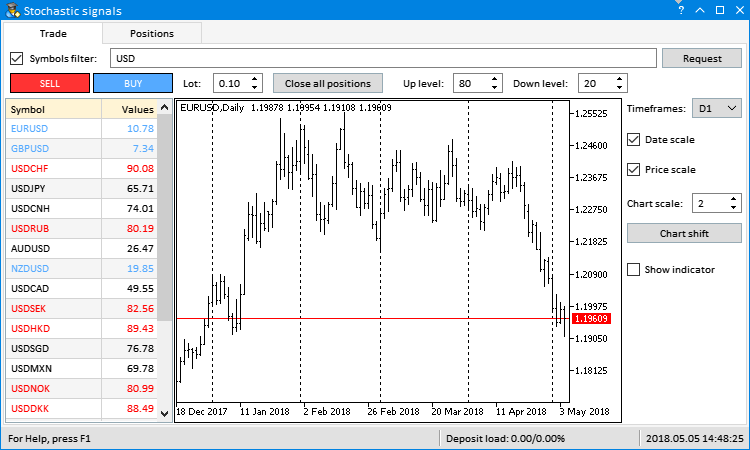Fig. 1. Initialized symbol table

If the account already has open positions at the moment the program is uploaded, the position table looks as follows: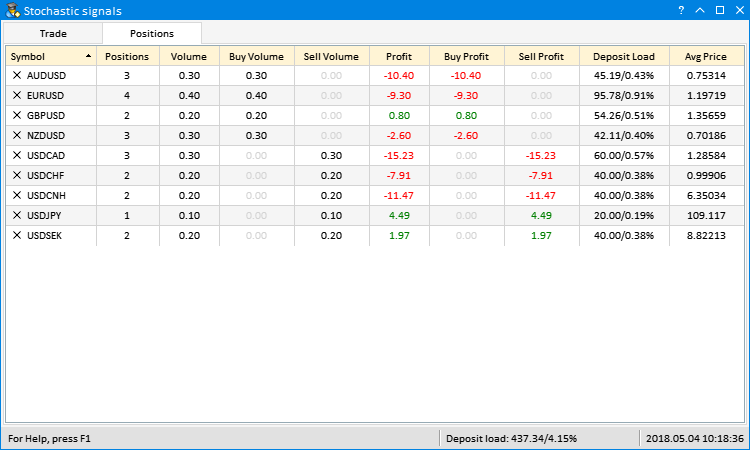Fig. 2. Initialized position table

### Updating tables in real time

The price is moving constantly, therefore the data in the tables should be constantly recalculated during a trading session. The table is to be updated at certain intervals set in the program timer. To provide the update of elements with different intervals, we can use CTimeCounter type objects. This class can be found in the EasyAndFast library. To use it in the project, simply include the file with its contents:

```//+------------------------------------------------------------------+
//|                                                      Program.mqh |
//|                        Copyright 2018, MetaQuotes Software Corp. |
//|                                              http://www.mql5.com |
//+------------------------------------------------------------------+
...
#include <EasyAndFastGUI\TimeCounter.mqh>
...```

Our EA needs three time counters for updating data in the progress bar and in the tables.

• The second and the third points are updated in the status bar by the timer. The second one displays the deposit load for the entire account, while the third one shows the current time of the trading server. Set the time interval for this counter to 500 ms.
• The current indicator values for all symbols are to be updated in the symbol table. Since there may be plenty of symbols, it is not recommended to do that too frequently, thus set the interval to 5000 ms.
• The position table features parameters depending on the current prices. Therefore, we need to update from time to time here as well to have relevant data. Let's set the interval of 1000 ms for this counter.

To set the counters, simply declare CTimeCounter type objects and set their parameters in the constructor (see the listing below). The first parameter is a timer frequency, while the second one is a time interval. The CTimeCounter::CheckTimeCounter() method returns 'true' after passing it. After that, the counter resets and starts counting anew.

```//+------------------------------------------------------------------+
//| Class for creating the application                               |
//+------------------------------------------------------------------+
class CProgram : public CWndEvents
{
private:
...
//--- Time counters
CTimeCounter      m_counter1;
CTimeCounter      m_counter2;
CTimeCounter      m_counter3;
...
};
//+------------------------------------------------------------------+
//| Constructor                                                      |
//+------------------------------------------------------------------+
CProgram::CProgram(void)
{
//--- Set parameters for time counters
m_counter1.SetParameters(16,500);
m_counter2.SetParameters(16,5000);
m_counter3.SetParameters(16,1000);
...
}```

Add the code displayed below to update the status bar in the first counter block of the program timer. To display the implemented changes, do not forget to update each point separately.

```//+------------------------------------------------------------------+
//| Timer                                                            |
//+------------------------------------------------------------------+
void CProgram::OnTimerEvent(void)
{
...
//--- Update points in the status bar
if(m_counter1.CheckTimeCounter())
{
//--- Set the values
//--- Update the points
m_status_bar.GetItemPointer(1).Update(true);
m_status_bar.GetItemPointer(2).Update(true);
}
...
}```

To accelerate the update of the table where only the indicator values should be updated, we will use the separate method — CProgram::UpdateSymbolsTable(). Before calling it, you should first update the indicator values array. The CProgram::UpdateSymbolsTable() method is called afterwards. The check for array out of range is performed here at each iteration. If the check is passed, update the cells of the second table column and adjust the text color. Receiving data and initializing the tables are displayed in the progress bar.

```//+------------------------------------------------------------------+
//| Update the symbol table                                          |
//+------------------------------------------------------------------+
{
uint values_total=::ArraySize(m_values);
//--- Add the values to the symbol table
uint rows_total=m_table_symb.RowsTotal();
for(uint r=0; r<(uint)rows_total; r++)
{
//--- Stop the loop in case of an array out of range
if(r>values_total-1 || values_total<1)
break;
//--- Set the values
m_table_symb.SetValue(1,r,::DoubleToString(m_values[r],2));
//--- Set the colors
color clr=(m_values[r]>(double)m_up_level.GetValue())? clrRed :(m_values[r]<(double)m_down_level.GetValue())? C'85,170,255' : clrBlack;
m_table_symb.TextColor(0,r,clr,true);
m_table_symb.TextColor(1,r,clr,true);
//--- Update the progress bar
m_progress_bar.LabelText("Initialize tables: "+string(rows_total)+"/"+string(r)+"...");
m_progress_bar.Update(r,rows_total);
::Sleep(5);
}
//--- Update the table
m_table_symb.Update();
}```

The block of the second time counter for updating the table is shown below. Thus, the program will receive the current values of the indicator for all symbols and update the table every 5 seconds.

```void CProgram::OnTimerEvent(void)
{
...
//--- Update the symbol table
if(m_counter2.CheckTimeCounter())
{
//--- Show the progress
m_progress_bar.Show();
m_chart.Redraw();
//--- Update the table values
GetIndicatorValues();
//--- Hide the progress
m_progress_bar.Hide();
m_chart.Redraw();
}
...
}```

To update the position table in the timer, first we receive the array of open positions' symbols. Then, update the table using the relevant data. Sort it by the same column and direction as before the update. Apply to the table for displaying implemented changes.

```void CProgram::OnTimerEvent(void)
{
...
//--- Update the position table
if(m_counter3.CheckTimeCounter())
{
//--- Get symbols of open positions
string symbols_name[];
int symbols_total=GetPositionsSymbols(symbols_name);
//--- Update the values in the table
SetValuesToPositionsTable(symbols_name);
//--- Sort if already done by a user before the update
m_table_positions.SortData((uint)m_table_positions.IsSortedColumnIndex(),m_table_positions.IsSortDirection());
//--- Update the table
UpdatePositionsTable();
}
}```

### Processing control events

In this section, we will consider the methods of handling events generated when interacting with the graphical interface of our EA. We have already analyzed the CProgram::RequestData() method for receiving symbols and indicator data. If this is not the first initialization, the method is called when clicking the Request button at any moment during the program execution. When clicking the button, a custom event with the ON_CLICK_BUTTON ID is generated.

```void CProgram::OnEvent(const int id,const long &lparam,const double &dparam,const string &sparam)
{
...
//--- Button pressing events
if(id==CHARTEVENT_CUSTOM+ON_CLICK_BUTTON)
{
//--- Data request
if(RequestData(lparam))
return;
//---
return;
}
...
}```

The gif image below displays the following. The table features the list of forex symbols containing USD. Then, we quickly form the list of symbols containing EUR. To do this, enter "EUR" in the Symbols filter input field and click Request. If you want to see all symbols with USD and EUR available on the server, they should be comma-separated: "USD,EUR".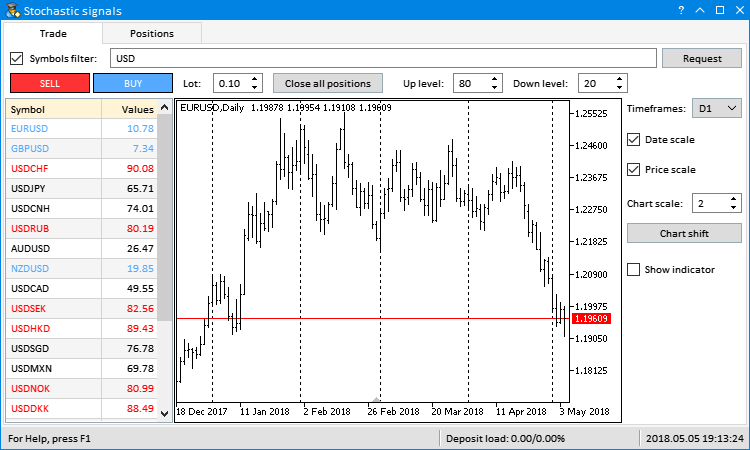Fig. 3. Forming the list of Forex symbols

Forming the list of forex symbols and getting the indicator handles is performed on the period specified in the Timeframes combo box. If we select another timeframe in the drop-down list, we should receive the new handles and update the table values. To achieve this, we need the CProgram::ChangePeriod() method. If the combo box ID has arrived, first update the timeframe in the object chart. Then, get the handles and indicator data for all table symbols. After that, the table is updated to display the implemented changes.

```//+------------------------------------------------------------------+
//| Change the timeframe                                             |
//+------------------------------------------------------------------+
bool CProgram::ChangePeriod(const long id)
{
//--- Check the element ID
if(id!=m_timeframes.Id())
return(false);
//--- Get the value from the combo box drop-down list
string tf=m_timeframes.GetListViewPointer().SelectedItemText();
//--- Get the chart pointer by index
m_sub_chart1.GetSubChartPointer(0).Period(StringToTimeframe(tf));
m_sub_chart1.ResetCharts();
//--- Show the progress
m_progress_bar.Show();
m_chart.Redraw();
//--- Get handles and indicator data
GetHandles();
GetIndicatorValues();
//--- Update the table
//--- Hide the progress
m_progress_bar.Hide();
m_chart.Redraw();
return(true);
}```

When selecting an item in the drop-down list, a custom event with the ON_CLICK_COMBOBOX_ITEM ID is generated:

```void CProgram::OnEvent(const int id,const long &lparam,const double &dparam,const string &sparam)
{
...
//--- Event of selecting an item in the combo box
if(id==CHARTEVENT_CUSTOM+ON_CLICK_COMBOBOX_ITEM)
{
//--- Change the timeframe
if(ChangePeriod(lparam))
return;
//---
return;
}
...
}```

Here is how changing the timeframe and receiving new data looks like: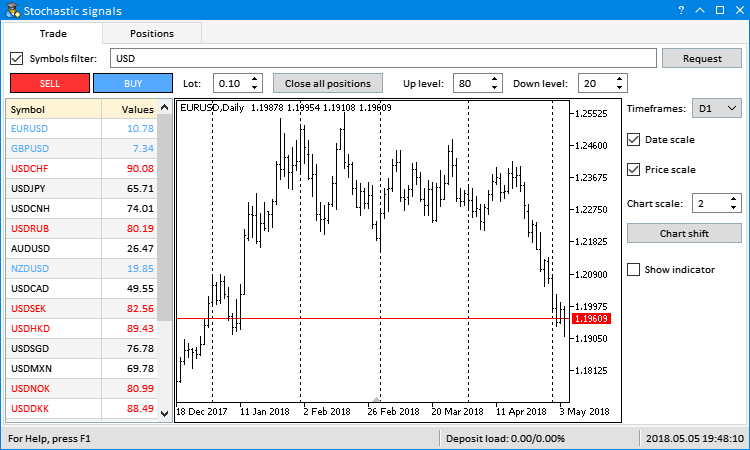Fig. 4. Changing the timeframe

Now, let's consider how to quickly change a symbol on an object chart. Symbol names are already present in the first column of the symbol table. Therefore, it is possible to switch between them by simply highlighting the table rows. The CProgram::ChangeSymbol() method is called by clicking on a necessary row. The symbol table ID is first checked here. Now, check if the table row is highlighted, since row highlighting is disabled by a repeated click. If checks are passed, save the highlighted row index as a handle one. It then can be used to place the indicator on a chart (considered below).

After receiving a symbol from the first table column using a highlighted row index, set it in the object chart. The full symbol description is displayed in the first section of the status bar as an additional info. When disabling the table row highlight, the text is changed for the default one.

```//+------------------------------------------------------------------+
//| Change the symbol                                                |
//+------------------------------------------------------------------+
bool CProgram::ChangeSymbol(const long id)
{
//--- Check the element ID
if(id!=m_table_symb.Id())
return(false);
//--- Exit if the row is not highlighted
if(m_table_symb.SelectedItem()==WRONG_VALUE)
{
//--- Show the full symbol description in the status bar
m_status_bar.SetValue(0,"For Help, press F1");
m_status_bar.GetItemPointer(0).Update(true);
return(false);
}
//--- Save the handle index
m_current_handle_index=m_table_symb.SelectedItem();
//--- Get the symbol
string symbol=m_table_symb.GetValue(0,m_current_handle_index);
//--- Update the chart
m_sub_chart1.GetSubChartPointer(0).Symbol(symbol);
m_sub_chart1.ResetCharts();
//--- Display the full symbol description in the status bar
m_status_bar.SetValue(0,::SymbolInfoString(symbol,SYMBOL_DESCRIPTION));
m_status_bar.GetItemPointer(0).Update(true);
m_chart.Redraw();
return(true);
}```

When highlighting a table row, a custom event with an ON_CLICK_LIST_ITEM ID is generated. Symbols can also be changed by Up, Down, Home and End keys. In this case, the CHARTEVENT_KEYDOWN event is generated. Its handling method was considered in the previous article, so there is no point to dwell on it here.

```void CProgram::OnEvent(const int id,const long &lparam,const double &dparam,const string &sparam)
{
...
//--- Event of selecting an item in the list/table
if(id==CHARTEVENT_CUSTOM+ON_CLICK_LIST_ITEM)
{
//--- Change the symbol
if(ChangeSymbol(lparam))
return;
//---
return;
}
//--- Click the button
if(id==CHARTEVENT_KEYDOWN)
{
//--- Select results using keys
if(SelectingResultsUsingKeys(lparam))
return;
//---
return;
}
...
}```

As a result of handling these events, we will see something like this: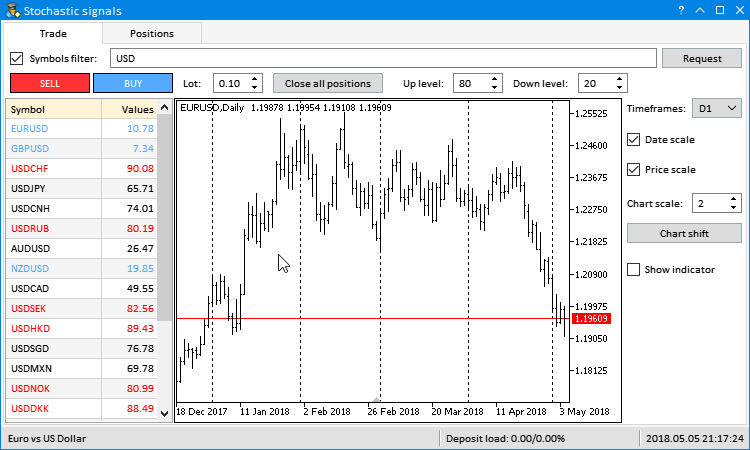Fig. 5. Switching symbols

Sometimes, we need to see the indicator used to get signals. The Show indicator check box allows you to enable it. The CProgram::ShowIndicator() method is responsible for interacting with it. Checks for an element ID and out of the handle array range should be passed here as well. A chart ID is needed to add or remove the indicator from the appropriate object chart. Then, if the check-box is enabled, add the indicator to the chart. Since we use a single indicator at all times, subwindow index is set to 1. For more complex cases, the number of indicators on a chart should be defined.

```//+------------------------------------------------------------------+
//| Indicator visibility                                             |
//+------------------------------------------------------------------+
bool CProgram::ShowIndicator(const long id)
{
//--- Check the element ID
if(id!=m_show_indicator.Id())
return(false);
//--- Check for array out of range
int handles_total=::ArraySize(m_handles);
if(m_current_handle_index<0 || m_current_handle_index>handles_total-1)
return(true);
//--- Get the chart ID
long sub_chart_id=m_sub_chart1.GetSubChartPointer(0).GetInteger(OBJPROP_CHART_ID);
//--- Subwindow index for the indicator
int subwindow =1;
//--- Get the chart pointer by index
if(m_show_indicator.IsPressed())
{
//--- Add the indicator to the chart
}
else
{
//--- Remove the indicator from the chart
::ChartIndicatorDelete(sub_chart_id,subwindow,ChartIndicatorName(sub_chart_id,subwindow,0));
}
//--- Update the chart
m_chart.Redraw();
return(true);
}```

When interacting with the check box, the ON_CLICK_CHECKBOX custom event is generated:

```void CProgram::OnEvent(const int id,const long &lparam,const double &dparam,const string &sparam)
{
...
//--- Event of clicking the "Check box" element
if(id==CHARTEVENT_CUSTOM+ON_CLICK_CHECKBOX)
{
//--- If clicked the "Show indicator" check box
if(ShowIndicator(lparam))
return;
//---
return;
}
}```

This is how it looks in actual practice: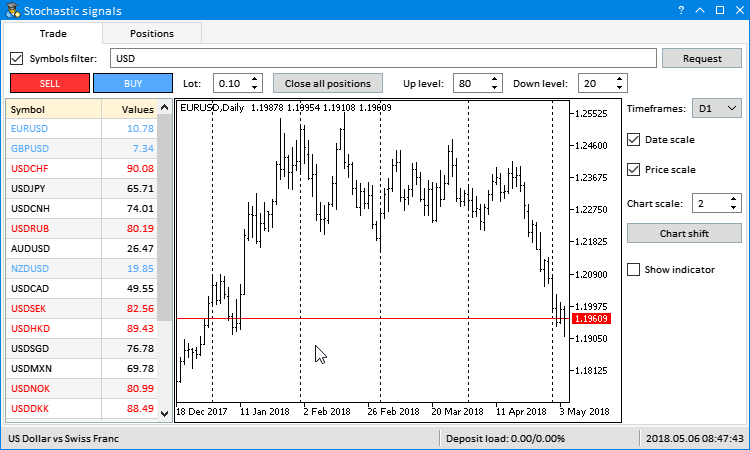Fig. 6. Displaying the indicator

Another two controls in the EA's GUI are also related to the indicator. These are digital input fields for the Stochastic indicator: Up level and Down level. By default, they are set to 80 and 20. If indicator values exceed these limits upwards and downwards at each symbol, the text in the symbol table cells is changed from black to blue for the upper level and to red — for the lower one. If we change the values in these input fields, the color indication changes as well (every five seconds) during the next update.

Here is how it works when you change values ​​from 80/20 to 90/10 and back: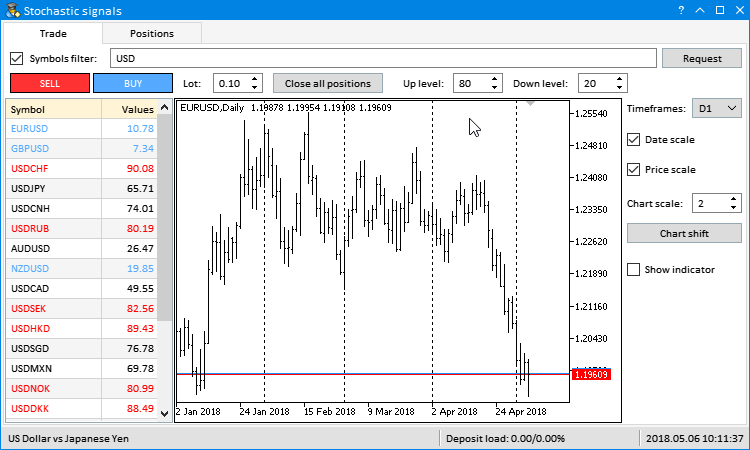Fig. 7. Changing the indicator signal levels

Several controls are meant for working with chart properties. These are:

• Date scale and Price scale check boxes for managing the visibility of the chart scales;
• Chart scale entry field for managing the chart scale
• and the Chart shift button for enabling the indent of the chart's right side.

Methods of handling events from the Date scale and Price scale check boxes are very similar. In both cases, an appropriate chart property is enabled or disabled depending on the check box status. The CStandardChart::ResetCharts() method moves the chart to the very end.

```//+------------------------------------------------------------------+
//| Time scale visibility                                            |
//+------------------------------------------------------------------+
bool CProgram::DateScale(const long id)
{
//--- Check the element ID
if(id!=m_date_scale.Id())
return(false);
//--- Get the chart pointer by index
m_sub_chart1.GetSubChartPointer(0).DateScale(m_date_scale.IsPressed());
m_sub_chart1.ResetCharts();
//--- Update the chart
m_chart.Redraw();
return(true);
}
//+------------------------------------------------------------------+
//| Price scale visibility                                           |
//+------------------------------------------------------------------+
bool CProgram::PriceScale(const long id)
{
//--- Check the element ID
if(id!=m_price_scale.Id())
return(false);
//--- Get the chart pointer by index
m_sub_chart1.GetSubChartPointer(0).PriceScale(m_price_scale.IsPressed());
m_sub_chart1.ResetCharts();
//--- Update the chart
m_chart.Redraw();
return(true);
}```

The CProgram::ChartScale() method is used to manage the chart scale. Here, if the value in the input field has changed, it is assigned to the chart.

```//+------------------------------------------------------------------+
//| Chart scale                                                      |
//+------------------------------------------------------------------+
bool CProgram::ChartScale(const long id)
{
//--- Check the element ID
if(id!=m_chart_scale.Id())
return(false);
//--- Set the scale
if((int)m_chart_scale.GetValue()!=m_sub_chart1.GetSubChartPointer(0).Scale())
m_sub_chart1.GetSubChartPointer(0).Scale((int)m_chart_scale.GetValue());
//--- Update
m_chart.Redraw();
return(true);
}```

Changing the value in the Chart scale input field is handled upon the arrival of custom events with ON_CLICK_BUTTON and ON_END_EDIT IDs.

```void CProgram::OnEvent(const int id,const long &lparam,const double &dparam,const string &sparam)
{
...
//--- Buttons clicking event
if(id==CHARTEVENT_CUSTOM+ON_CLICK_BUTTON)
{
//--- Chart scale
if(ChartScale(lparam))
return;
//---
return;
}
//--- Event of finishing changing the value in the input field
if(id==CHARTEVENT_CUSTOM+ON_END_EDIT)
{
//--- Chart scale
if(ChartScale(lparam))
return;
//---
return;
}
}```

The CProgram::ChartShift() method code for enabling the right indent in the chart is shown below. After checking for the element ID, get the chart ID, and use it as an access key to set the indent (CHART_SHIFT).

```//+------------------------------------------------------------------+
//| Chart shift                                                      |
//+------------------------------------------------------------------+
bool CProgram::ChartShift(const long id)
{
//--- Check the element ID
if(id!=m_chart_shift.Id())
return(false);
//--- Get the chart ID
long sub_chart_id=m_sub_chart1.GetSubChartPointer(0).GetInteger(OBJPROP_CHART_ID);
//--- Set the indent in the right part of the chart
::ChartSetInteger(sub_chart_id,CHART_SHIFT,true);
m_sub_chart1.ResetCharts();
return(true);
}```

Here is how it looks: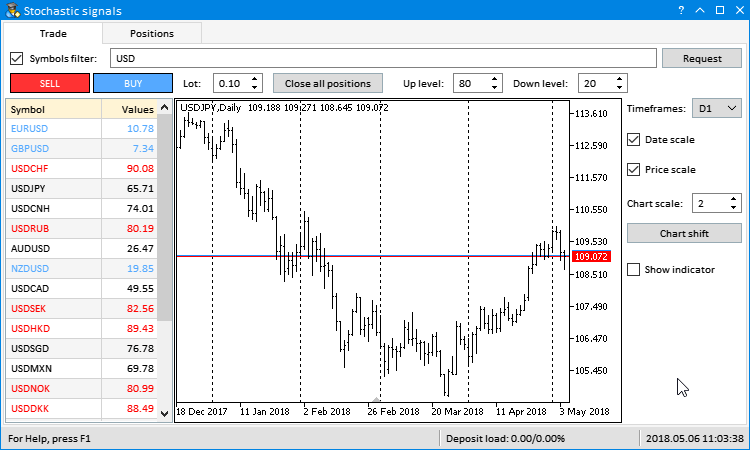Fig. 8. Managing the chart properties

### Methods of performing trade operations

I will use examples to show you how to quickly link trading methods with the EA's GUI. Our EA will not only visualize data but also perform trading operations. It is more convenient to work when everything is in one place and you can quickly switch charts and trade if necessary. As an example, we will use the standard library features for trading operations. Other trading libraries can be included as well.

Include the Trade.mqh file with the CTrade class to the project and declare the instance of the class:

```//--- Class for trading operations
//+------------------------------------------------------------------+
//| Class for creating applications                                  |
//+------------------------------------------------------------------+
class CProgram : public CWndEvents
{
private:
};```

Set asynchronous trading mode, so that the program does not have to wait for the result of each trade operation. Besides, set the maximum allowable slippage. This means the trades are performed at any deviation from the price specified in a trade operation.

```//+------------------------------------------------------------------+
//| Constructor                                                      |
//+------------------------------------------------------------------+
CProgram::CProgram(void)
{
...
}```

Clicking on Buy and Sell buttons are to be handled by similar methods — CProgram::OnBuy() and CProgram::OnSell(). A deal volume is received from the Lot input field. A traded symbol is to be taken in the object chart. This is the minimum set of actions required to complete a trade operation. The CTrade class features the CTrade::Buy() and CTrade::Sell() methods. Only two of these arguments can be passed when calling the methods.

```//+------------------------------------------------------------------+
//+------------------------------------------------------------------+
{
//--- Check the element ID
return(false);
//--- Volume and symbol for opening a position
double lot    =::NormalizeDouble((double)m_lot.GetValue(),2);
string symbol =m_sub_chart1.GetSubChartPointer(0).Symbol();
//--- Open a position
return(true);
}
//+------------------------------------------------------------------+
//| Selling                                                          |
//+------------------------------------------------------------------+
bool CProgram::OnSell(const long id)
{
//--- Check the element ID
if(id!=m_sell.Id())
return(false);
//--- Volume and symbol for opening a position
double lot    =::NormalizeDouble((double)m_lot.GetValue(),2);
string symbol =m_sub_chart1.GetSubChartPointer(0).Symbol();
//--- Open a position
return(true);
}```

A separate method should be implemented to close all positions simultaneously or by a specified symbol, since the CTrade class does not have one. If a symbol (optional parameter) is passed to the method, position on that symbol only are closed. If no symbol is specified, all positions are closed.

```//+------------------------------------------------------------------+
//| Close all positions                                              |
//+------------------------------------------------------------------+
bool CProgram::CloseAllPosition(const string symbol="")
{
//--- Check if a position with specified properties is present
int total=::PositionsTotal();
for(int i=total-1; i>=0; i--)
{
//--- Select a position
string pos_symbol=::PositionGetSymbol(i);
//--- If closing by a symbol
if(symbol!="")
if(symbol!=pos_symbol)
continue;
//--- Get the ticket
ulong position_ticket=::PositionGetInteger(POSITION_TICKET);
//--- Reset the last error
::ResetLastError();
//--- If the position has not closed, inform of this
::Print(__FUNCTION__,": > An error occurred when closing a position: ",::GetLastError());
}
//---
return(true);
}```

Closing all positions is bound to the "Close all positions" button. Its clicking is to be handled in the CProgram::OnCloseAllPositions() method. To avoid accidental clicks on the button, the confirmation window is to be opened.

```//+------------------------------------------------------------------+
//| Close all positions                                              |
//+------------------------------------------------------------------+
bool CProgram::OnCloseAllPositions(const long id)
{
//--- Check element ID
if(id!=m_close_all.Id())
return(false);
//--- Dialog window
int mb_id=::MessageBox("Are you sure you want to close \nall positions?","Close positions",MB_YESNO|MB_ICONWARNING);
//--- Close positions
if(mb_id==IDYES)
CloseAllPosition();
//---
return(true);
}```

This is how it looks: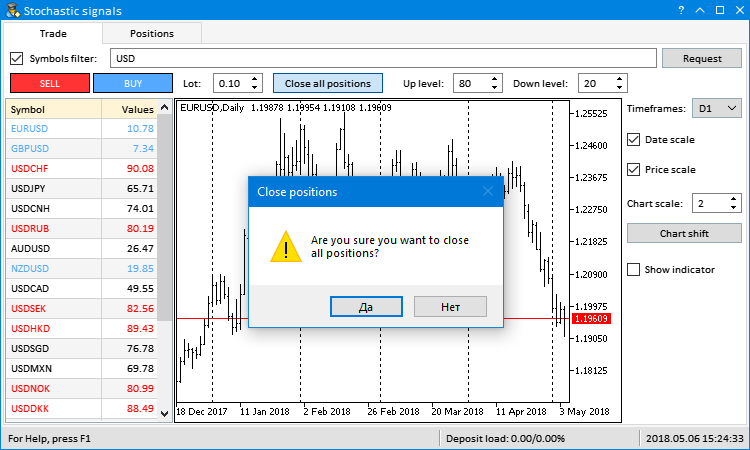Fig. 9. Closing all positions

Positions on a specified symbol can be closed on the Positions tab. The cross buttons are added in the cells of the position table's first column. They allow you to simultaneously close all positions on a symbol displayed in the appropriate row. Clicking the cell buttons generates a user event having the ON_CLICK_BUTTON ID. But the CTable type element has scroll bars, and their buttons generate the same events, while the element ID is the same. This means we need to track the string parameter (sparam) of an event to avoid accidental handling of a click on other buttons of the element. In the string parameter, we define the type of the element a click has occurred on. For scroll bars, this is the "scroll" value. If an event with such a value arrives, the program exits the method. After that, we need to check whether open positions are still present.

If all checks are passed, we need to extract the row index defining a symbol in the first table column from the string parameter description. To avoid accidental clicks on the buttons, the dialog window for confirming actions is opened first. Clicking Yes closes positions for a specified symbol only

```//+------------------------------------------------------------------+
//| Close all positions for a specified symbol                       |
//+------------------------------------------------------------------+
bool CProgram::OnCloseSymbolPositions(const long id,const string desc)
{
//--- Check the element ID
if(id!=m_table_positions.Id())
return(false);
//--- Exit if this is a click on the scroll bar button
if(::StringFind(desc,"scroll",0)!=WRONG_VALUE)
return(false);
//--- Exit if there are no positions
if(::PositionsTotal()<1)
return(true);
//--- Extract data from the string
string str_elements[];
ushort sep=::StringGetCharacter("_",0);
::StringSplit(desc,sep,str_elements);
//--- Get the index and the symbol
int    row_index =(int)str_elements;
string symbol    =m_table_positions.GetValue(0,row_index);
//--- Dialog window
int mb_id=::MessageBox("Are you sure you want to close \nall positions on symbol "+symbol+"?","Close positions",MB_YESNO|MB_ICONWARNING);
//--- Close all positions on a specified symbol
if(mb_id==IDYES)
CloseAllPosition(symbol);
//---
return(true);
}```

Here is how it looks: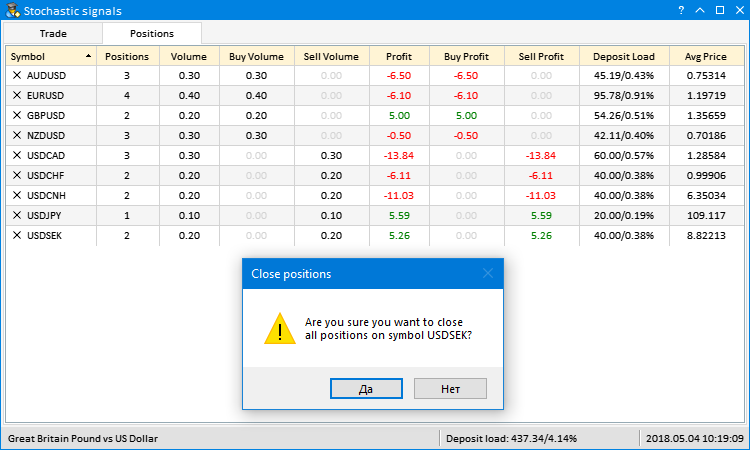Fig. 10. Close all positions on a specified symbol

All trading operations described above are handled upon the arrival of the ON_CLICK_BUTTON event:

```void CProgram::OnEvent(const int id,const long &lparam,const double &dparam,const string &sparam)
{
...
//--- Button clicking events
if(id==CHARTEVENT_CUSTOM+ON_CLICK_BUTTON)
{
...
return;
//--- Sell
if(OnSell(lparam))

return;
//--- Close all positions
if(OnCloseAllPositions(lparam))
return;
//--- Close all positions on a specified symbol
if(OnCloseSymbolPositions(lparam,sparam))
return;
//---
return;
}
...
}```

Each trading operation should be added to the position table. To achieve this, we need to track trading events and account's trading history. If the number of deals has changed, the table should be formed anew. The CProgram::IsLastDealTicket() method is used to check if the history has changed. Time and last deal ticket should be saved after each check. We save the time in order not to constantly request the entire history of deals. The ticket allows us to check if the number of deals in history changed. Since the deal initiates several trading events, this method returns true only once.

```class CProgram : public CWndEvents
{
private:
//--- Time and ticket of the last checked deal
datetime          m_last_deal_time;
ulong             m_last_deal_ticket;
//---
private:
//--- Check a new deal in history
bool              IsLastDealTicket(void);
};
//+------------------------------------------------------------------+
//| Constructor                                                      |
//+------------------------------------------------------------------+
CProgram::CProgram(void) : m_last_deal_time(NULL),
m_last_deal_ticket(WRONG_VALUE)
{
...
}
//+------------------------------------------------------------------+
//| Check a new deal in history                                      |
//+------------------------------------------------------------------+
bool CProgram::IsLastDealTicket(void)
{
//--- Exit if no history is received
if(!::HistorySelect(m_last_deal_time,UINT_MAX))
return(false);
//--- Get the number of deals in the obtained list
int total_deals=::HistoryDealsTotal();
//--- Go through all deals in the obtained list from the last deal to the first one
for(int i=total_deals-1; i>=0; i--)
{
//--- Get the deal ticket
ulong deal_ticket=::HistoryDealGetTicket(i);
//--- Exit if the tickets match
if(deal_ticket==m_last_deal_ticket)
return(false);
//--- If the tickets do not match, inform of this
else
{
datetime deal_time=(datetime)::HistoryDealGetInteger(deal_ticket,DEAL_TIME);
//--- Remember the last deal's time and ticket
m_last_deal_time   =deal_time;
m_last_deal_ticket =deal_ticket;
return(true);
}
}
//--- Another symbol's tickets
return(false);
}```

The CProgram::IsLastDealTicket() method is called in the trade event handler. If the history changed, the position table is formed anew:

```//+------------------------------------------------------------------+
//+------------------------------------------------------------------+
{
//--- In case of a new deal
if(IsLastDealTicket())
{
//--- Initialize the position table
InitializePositionsTable();
}
}```

Here is how it looks: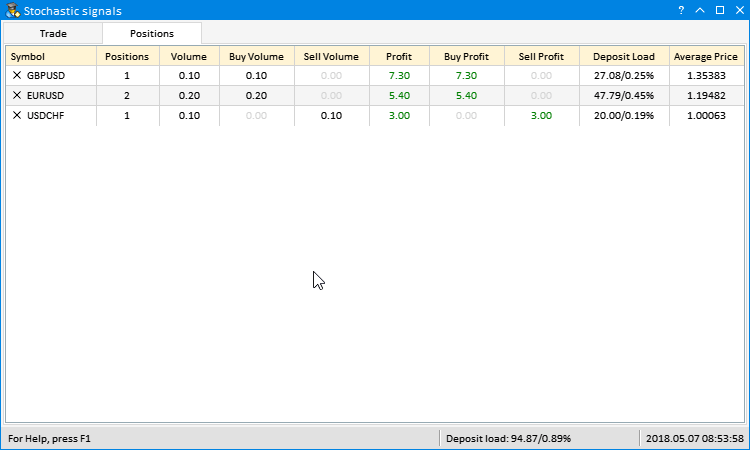Fig. 11. Forming the table when closing positions on a symbol

### Conclusion

We have discussed how to develop GUIs for programs of any complexity without excessive effort. You can continue to develop this program and use it for your own purposes. The idea can be improved by adding custom indicators and calculation results.

The attachments contain the files for testing and more detailed study of the code presented in the article.

File name Comment
MQL5\Experts\TradePanel\CreateGUI.mqh Methods for developing GUI from the program class in Program.mqh
MQL5\Include\EasyAndFastGUI\Controls\Table.mqh Updated CTable class
MQL5\Include\EasyAndFastGUI\Keys.mqh Updated CKeys class

Translated from Russian by MetaQuotes Software Corp.
Original article: https://www.mql5.com/ru/articles/4727

Attached files |
MQL5.zip (48 KB)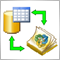Integrating MQL-based Expert Advisors and databases (SQL Server, .NET and C#)

The article describes how to add the ability to work with Microsoft SQL Server database server to MQL5-based Expert Advisors. Import of functions from a DLL is used. The DLL is created using the Microsoft .NET platform and the C# language. The methods used in the article are also suitable for experts written in MQL4, with minor adjustments.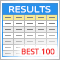Visualizing optimization results using a selected criterion

In the article, we continue to develop the MQL application for working with optimization results. This time, we will show how to form the table of the best results after optimizing the parameters by specifying another criterion via the graphical interface.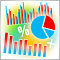Trading account monitoring is an indispensable trader's tool

Trading account monitoring provides a detailed report on all completed deals. All trading statistics are collected automatically and provided to you as easy-to-understand diagrams and graphs.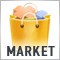14,000 trading robots in the MetaTrader Market

The largest store of ready-made applications for algo-trading now features 13,970 products. This includes 4,800 robots, 6,500 indicators, 2,400 utilities and other solutions. Almost half of the applications (6,000) are available for rent. Also, a quarter of the total number of products (3,800) can be downloaded for free.